# GSEB Solutions Class 11 Maths Chapter 10 Straight Lines Ex 10.2

Gujarat Board GSEB Textbook Solutions Class 11 Maths Chapter 10 Straight Lines Ex 10.2 Textbook Questions and Answers.

## Gujarat Board Textbook Solutions Class 11 Maths Chapter 10 Straight Lines Ex 10.2

In questions 1 to 7, find the equations of the line, which satisfy the given conditions:
1. Write the equations for x and y-axis.
2. passing through (- 4, 3) with slope $$\frac{1}{2}$$.
3. Passing through (0, 0) with slope m.
4. Passing through (2, 2$$\sqrt{3}$$) and inclined with the x-axis at an angle of 75°.
5. Intersecting the x-axis at a distance of 3 units to the left of origin with slope – 2.
6. Intersecting the y-axis at a distance of 2 units above the origin and making an angle of 30° with the positive direction of the x-axis.
7. Passing through the points (- 1, 1) and (2, – 4).
Solutions to questions 1 to 7:
1. Equation of the x-axis is y = 0.
Equation of the y-axis is x = 0.2. Equation of a line passing through (x1, y1) with slope m is (y – y1) = m(x – x1).
Here, x1 = – 4, y1 = 3, m = $$\frac{1}{2}$$.
∴ Equation of the required line is
(y – 3) = $$\frac{1}{2}$$ (x + 4)
or 2y – 6 = x + 4 or x – 2y + 10 = 0

3. x1 = 0, y1 = 0, Slope = m.
Equation of the required line is
(y – 0) = m(x – 0)
or y = mx.4. Here, x1 = 2, y1 = 2$$\sqrt{3}$$
m = tan 75° = tan (45° + 30°)
= $$\frac{tan 45° + tan 30°}{1 – tan 45° tan 30°}$$ [∵ tan(A + B) = $$\frac{tan A + tan B}{1 – tan A tan B}$$Equation of the required line is
y – 2$$\sqrt{3}$$ = $$\frac{\sqrt{3}+1}{\sqrt{3}-1}$$ (x – 2).
($$\sqrt{3}$$ – 1)y – 2$$\sqrt{3}$$($$\sqrt{3}$$ – 1) = ($$\sqrt{3}$$ + 1)x – 2($$\sqrt{3}$$ + 1)
or ($$\sqrt{3}$$ – 1)y – 6 + 2$$\sqrt{3}$$ = ($$\sqrt{3}$$ + 1)x – 2$$\sqrt{3}$$ – 2.
or ($$\sqrt{3}$$ + 1)x – ($$\sqrt{3}$$ – 1)y = – 6 + 2$$\sqrt{3}$$ + 2$$\sqrt{3}$$ + 2.
= 4$$\sqrt{3}$$ – 4 = 4($$\sqrt{3}$$ – 1).
∴ Then, equation of required line is
($$\sqrt{3}$$ + 1)x – ($$\sqrt{3}$$ – 1)y = 4($$\sqrt{3}$$ – 1).

5. The line AB meets x-axis at (- 3, 0) and slope of the line = – 2.
∴ Equation of the line is y – 0 = – 2(x + 3)
or 2x + y + 6 = 0.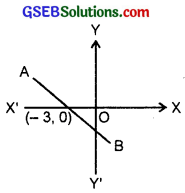6. The line PQ intersects y-axis at (0, 2).
Slope of PQ = tan 30° = $$\frac{1}{\sqrt{3}}$$
Equation of PQ is
y – 2 = $$\frac{1}{\sqrt{3}}$$(x – 0).
or $$\sqrt{3y}$$ – 2$$\sqrt{3}$$ = x
or x – $$\sqrt{3}$$y + 2$$\sqrt{3}$$ = 0.7. The line passes through the points A(- 1, 1) and B(2, – 4). Equation of the line passing through (x1, y1) and (x2, y2) is
y – y1 = $$\frac{y_{2}-y_{1}}{x_{2}-x_{1}}$$(x – x1)
Here, x1 = – 1, y1 = 1, x2 = 2, y2 = – 4.
∴ Equation of AB is
y – 1 = $$\frac{-4-1}{2+1}$$(x + 1) = $$\frac{-5}{3}$$(x + 1).
or 3(y – 1) = – 5(x + 1).
or 3y – 3 = – 5x – 5.
or 5x + 3y – 3 + 5 = 0
or 5x + 3y + 2 = 0.Question 8.
Find the equation of a line whose perpendicular distance from the origin is 5 units and the angle made by the perpendicular with positive x-axis is 30°.
Solution:
If a line is at a distance p from the origin and perpendicular makes an angle to with positive direction of x-axis, its equation is
xcos ω + ysin ω – p.
Here, p = 5, ω = 30°.
∴ Equation of the required line is
x cos 30° + y sin 30° = 5.
or $$\frac{\sqrt{3}}{2}$$x + $$\frac{1}{2}$$y = 5
or $$\sqrt{3x}$$ + y = 10.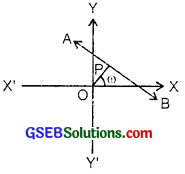Question 9.
The vertices of a triangle PQR are P(2, 1), Q(- 2, 3) and R(4, 5). Find the equation of the median through the vertex R.
Solution:
The vertices P and Q are (2,1) and (- 2, 3).
The middle point of PQ is S ($$\frac{2-2}{0}$$, $$\frac{1+3}{2}$$) or S(0, 2).
∴ Equation of the median RS, where R is (4, 5) and S is the point (0, 2) is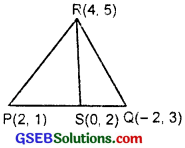y – 5 = $$\frac{2-5}{0-4}$$(x – 4).
[Apply formula y – y1 = $$\frac{y_{2}-y_{1}}{x_{2}-x_{1}}$$(x – x1)
or y – 5 = $$\frac{-3}{-4}$$(x – 4).
or 4(y – 5) = 3(x – 4) or 4y – 20 = 3x – 12.
i.e; 3x – 4y = – 20 + 12 – 8
∴ Equation of median RS is 3x – 4y + 8 = 0.Question 10.
Find the equation of the line passing through the point (- 3, 5) and perpendicular to the line through the points (2, 5) and (- 3, 6).
Solution:
Slope of the line joining A(2, 5) and B(- 3, 6) = $$\frac{6-5}{-3-2}$$ = $$\frac{1}{-5}$$.
∴ m = slope of any line ⊥ to AB = 5 [m1 = $$\frac{-1}{m_{2}}$$.
The equation of the line passing through (- 3, 5) and perpendicular to AB is
y – 5 = 5(x + 3)
⇒ 5x – y + 20 = 0.

Question 11.
A line perpendicular to the line segment joining the points (1, 0) and (2, 3) divides it in the ratio 1 : n. Find the equation of the line.
Solution:
Slope of the line joining the points A(1, 0) and B(2, 3)
= $$\frac{3-0}{2-1}$$ = $$\frac{3}{1}$$ = 3.∴ Slope of the CD perpendicular to AB = – $$\frac{1}{3}$$ [The lines are ⊥, so m1m2 = – 1]
The point P divides AB in the ratio 1 : n.
∴ Coordinates of P are
($$\frac{1×2+1×n}{1+n}$$, $$\frac{1×3+0×n}{1+n}$$) or ($$\frac{n+2}{n+1}$$, $$\frac{3}{n+1}$$).
∴ Equation of the line CD which is ⊥ to AB and passes through P is
y – $$\frac{3}{n+1}$$ = – $$\frac{1}{3}$$(x – $$\frac{n+2}{n+1}$$)
[Using the formula y – y1 = m(x – x1)]
or 3(n + 1)y – 9 = – (n + 1) (x – $$\frac{n+2}{n+1}$$)
= – (n + 1)x + (n + 2)
or (n + 1)x + 3(n + 1)y = n + 2 + 9
or (n + 1)x + 3(n + 1)y = r + 11.Question 12.
Find the equation of a line that cuts off equal intercepts on the co-ordinate axes and passes through (2, 3).
Solution:
The line making equal intercepts has the slope either 1 or – 1.
∴ tan 45° – 1
or tan 135° = – 1.
Now(i) slope = 1, P(2, 3)
∴ Equatirn of the line PR of slope 1 and passing through P(2, 3) is
y – 3 – 1(x – 2)
[Using the formula y – y1 = m(x – x1)]
or x – y + 1 = 0.(ii) When the slope = – 1.
Equation of the lire with slope – 1 and passing through P(2, 3) is
y – 3 = – 1 × (x – 2).
or x + y – 3 – 2 = 0,
or x + y – 5 = 0.Question 13.
Find the equations of the lines passing through the point (2, 2), such that the sum of their intercepts on the axes is 9.
Solution:
Let the intercepts along x-axis and y-axis be a and b.
∴ a + b = 9.
or b = 9 – a …………….. (1)
∴ Equation of the line in intercepts form is
$$\frac{x}{a}$$ + $$\frac{y}{b}$$ = 1 ………………….. (2)
⇒ $$\frac{x}{a}$$ + $$\frac{y}{9-a}$$ = 1 [Using (1)]
Since point A(2, 2) lies on it, therefore
$$\frac{2}{a}$$ + $$\frac{2}{9-a}$$ = 1.
⇒ $$\frac{2(9-a)+2a}{a(9-a)}$$ = 1.
⇒ 18 – 2a + 2a = a(9 – a)
⇒ 18 = 9a – a2
⇒ a2 – 9a + 18 = 0 ⇒ (a – 3)(a – 6) = 0.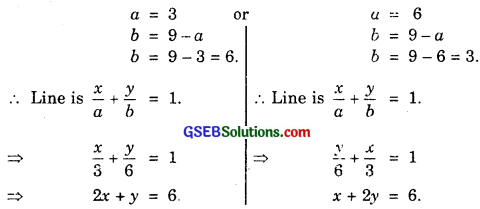Hence, the required equations of straight lines are 2x + y = 6 and x + 2y = 6.Question 14.
Find the equation of the line through the point (0, 2) making an angle $$\frac{2π}{3}$$ with the positive x-axis. Also, find the equation of the line parallel to it and crossing the y-axis at a distance of 2 units below the origin.
Solution:
Slope of the line = tan $$\frac{2π}{3}$$
= tan (π – $$\frac{π}{3}$$)
= – $$\frac{π}{3}$$ = – $$\sqrt{3}$$.Equation of the line AC passing through (0, 2) and having the slope – $$\sqrt{3}$$ is
y – 2 = – $$\sqrt{3}$$ (x – 0).
[Using the formula y – y1 = m(x – x1)]
⇒ $$\sqrt{3}$$x + y – 2 = 8.
Another line BD is parallel to AC.
∴ Slope of BD = Slope of AC = – $$\sqrt{3}$$.
BD is passing through the point B(0, – 2).
∴ Equation of BD is
y + 2 = – $$\sqrt{3}$$(x – 0).
or $$\sqrt{3x}$$ + y + 2 = 0.
Thus, equations of AC and BD are
$$\sqrt{3x}$$ + y – 2 = 0 and $$\sqrt{3x}$$ + y + 2 = 0.Question 15.
The perpendicular from the origin to a line meets at the point (- 2, 9). Find the equation of the line.Solution:
Let ON be the perpendicular to AB.
The point N is (- 2, 9).
∴ Slope of ON = $$\frac{9-0}{-2-0}$$ = $$\frac{- 9}{2}$$ [Slope = $$\frac{y_{2}-y_{1}}{x_{2}-x_{1}}$$]
Slope of AB which is ⊥ ON = $$\frac{2}{9}$$ [∵ Lines are ⊥ if m1m2 = – 1]
Now AB passes through (- 2, 9) and has the slope $$\frac{2}{9}$$
∴ Equation of AB is y – 9 = $$\frac{2}{9}$$(x + 2) [y – y1 = m(x – x1)]
or 9y – 81 = 2x + 4
or 2x – 9y + 85 = 0.

Question 16.
The length L (in centimetres) of a copper rod is a linear function of its Celsius temperature C. In an experiment, if L = 124.942, when C = 20 and L = 125.134, when C = 110, express L in terms of C.
Solution:
L is a linear function of C.
∴ L = a + bC.
For L = 124.942, C = 20.
∴ 124.942 = a + 20b …………….. (1)
For L = 125.134, C = 110.
∴ 125.134 = a + 110b …………….. (2)
Subtracting (1) from (2), we get
0.192 = 90b.
∴ b = $$\frac{0.192}{90}$$ = 0.00213.
From (1), 124.942 = a + 20 × 0.00213
= a + 0.0426.
∴ a = 124.942 – 0.0426
= 124.8994.
Now, L in terms of C is
L = a + bC
⇒ L = 124.8994 + 0.00213CQuestion 17.
The owner of a milk store finds that he can sell 980 litres of milk each week at ₹ 14/litre and 1220 litres of milk each week at ₹ 16/litre. Assuming a linear relationship between selling price and demand, how many litres could he sell weekly at ₹ 17/litre.
Solution:
Let y litres milk is sold at ₹ x/litre.
x and y have linear relationship, i.e.,
y = a + bx, i.e., it is a straight line.
Now, y1 = 980 litres, x1 = ₹ 14/litre.
y2 = 1220 litres, x2 = ₹ 16/litre.
Slope of the line = $$\frac{1220 – 980}{16 – 14}$$ = $$\frac{240}{2}$$ = 120.
∴ Equation of the line is
y – 980 = 120(x – 14).
When x = 17, y = 980 + 120(17 – 14)
= 980 + 120 × 3
= 980 + 360 = 1340.
Hence, 1340 litres milk may be sold at ₹ 17/litre.Question 18.
P(a, b) is the mid-point of a line segment between axes. Show that the equation of the corresponding line is $$\frac{x}{a}$$ + $$\frac{x}{b}$$ = 2.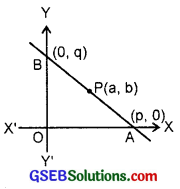Solution:
Let the line AB makes intercepts p and q on the axes.
∴ A is (p, 0) and B is (0, q).
Now, P(a, b) is the mid-point of AB.
∴ $$\frac{p+0}{2}$$ = a, $$\frac{0+q}{2}$$ = b.
∴ p = 2a, q = 2b.
Intercepts form of the line AB
$$\frac{x}{p}$$ + $$\frac{x}{q}$$ = 1.
or $$\frac{x}{2a}$$ + $$\frac{y}{2b}$$ = 1 or $$\frac{x}{a}$$ + $$\frac{y}{b}$$ = 2.

Question 19.
Point (h, k) divides a line segment between the axes in the ratio 1 : 2. Find equation of the corresponding line.
Solution:
Let the line AB makes intercepts a and b on the axes. The point R(h, k) divides line segment AB in the ratio 1 : 2.
So, h = $$\frac{1×0+2×a}{1+2}$$
= $$\frac{2a}{3}$$
∴ a = $$\frac{3}{2}$$h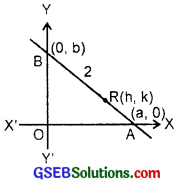and k = $$\frac{1×b+2×0}{1+2}$$ = $$\frac{b}{3}$$.
∴ b = 3k.
Intercepts form of the line AB is
$$\frac{x}{a}$$ + $$\frac{y}{b}$$ = 1.
or $$\frac{x}{3/2h}$$ + $$\frac{y}{3k}$$ = 1 or $$\frac{2x}{3h}$$ + $$\frac{y}{3k}$$ = 1.
or 2kx + hy = 3kh.Question 20.
By using the concept of equation of a line, prove that the three points (3, 0), (- 2, – 2) and (8, 2) are collinear.
Solution:
Equation of the line passing through (3, 0) and (- 2, – 2) is
y – 0 = $$\frac{-2-0}{-2-3}$$(x – 3)
[Apply y – y1 = $$\frac{y_{2}-y_{1}}{x_{2}-x_{1}}$$(x – x1)
= $$\frac{2}{5}$$(x – 3)
or 5y = 2x – 6 or 2x – 5y = 6.
If the point (8, 2) lies on it, (8, 2) will satisfy the equation of the line.
∴ 2 × 8 – 5 × 2
= 16 – 10 = 6.
Hence, (8, 2) lies on it.
Thus, (3, 0), (- 2, – 2) and (8, 2) are collinear.